# Manifold

Manifold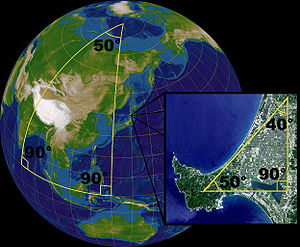The sphere (surface of a ball) is a two-dimensional manifold since it can be represented by a collection of two-dimensional maps.

In mathematics (specifically in differential geometry and topology), a manifold is a topological space that on a small enough scale resembles the Euclidean space of a specific dimension, called the dimension of the manifold. Thus, a line and a circle are one-dimensional manifolds, a plane and sphere (the surface of a ball) are two-dimensional manifolds, and so on into high-dimensional space. More formally, every point of an n-dimensional manifold has a neighborhood homeomorphic to an open subset of the n-dimensional space Rn.

Although manifolds resemble Euclidean spaces near each point ("locally"), the global structure of a manifold may be more complicated. For example, any point on the usual two-dimensional surface of a sphere is surrounded by a circular region that can be flattened to a circular region of the plane, as in a geographical map. However, the sphere differs from the plane "in the large": in the language of topology, they are not homeomorphic. The structure of a manifold is encoded by a collection of charts that form an atlas, in analogy with an atlas consisting of charts of the surface of the Earth.

The concept of manifolds is central to many parts of geometry and modern mathematical physics because it allows more complicated structures to be expressed and understood in terms of the relatively well-understood properties of simpler spaces. For example, a manifold is typically endowed with a differentiable structure that allows one to do calculus and a Riemannian metric that allows one to measure distances and angles. Symplectic manifolds serve as the phase spaces in the Hamiltonian formalism of classical mechanics, while four-dimensional Lorentzian manifolds model space-time in general relativity.

## Motivational examples

### Circle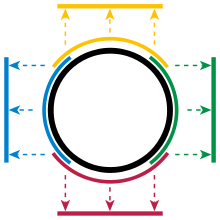Figure 1: The four charts each map part of the circle to an open interval, and together cover the whole circle.

After a line, the circle is the simplest example of a topological manifold. Topology ignores bending, so a small piece of a circle is treated exactly the same as a small piece of a line. Consider, for instance, the top half of the unit circle, x2 + y2 = 1, where the y-coordinate is positive (indicated by the yellow arc in Figure 1). Any point of this semicircle can be uniquely described by its x-coordinate. So, projection onto the first coordinate is a continuous, and invertible, mapping from the upper semicircle to the open interval (−1,1):$\chi_{\mathrm{top}}(x,y) = x . \,$

Such functions along with the open regions they map are called charts. Similarly, there are charts for the bottom (red), left (blue), and right (green) parts of the circle. Together, these parts cover the whole circle and the four charts form an atlas for the circle.

The top and right charts overlap: their intersection lies in the quarter of the circle where both the x- and the y-coordinates are positive. The two charts χtop and χright each map this part into the interval (0, 1). Thus a function T from (0, 1) to itself can be constructed, which first uses the inverse of the top chart to reach the circle and then follows the right chart back to the interval. Let a be any number in (0, 1), then:\begin{align} T(a) &= \chi_{\mathrm{right}}\left(\chi_{\mathrm{top}}^{-1}\left[a\right]\right) \\ &= \chi_{\mathrm{right}}\left(a, \sqrt{1-a^2}\right) \\ &= \sqrt{1-a^2} \end{align}

Such a function is called a transition map.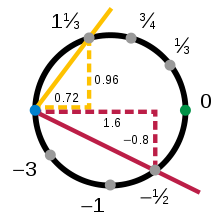Figure 2: A circle manifold chart based on slope, covering all but one point of the circle.

The top, bottom, left, and right charts show that the circle is a manifold, but they do not form the only possible atlas. Charts need not be geometric projections, and the number of charts is a matter of some choice. Consider the charts$\chi_{\mathrm{minus}}(x,y) = s = \frac{y}{1+x}$

and$\chi_{\mathrm{plus}}(x,y) = t = \frac{y}{1-x}{}$

Here s is the slope of the line through the point at coordinates (x,y) and the fixed pivot point (−1, 0); t is the mirror image, with pivot point (+1, 0). The inverse mapping from s to (xy) is given by\begin{align} x &= \frac{1-s^2}{1+s^2} \\ y &= \frac{2s}{1+s^2} \end{align}

It can easily be confirmed that x2 + y2 = 1 for all values of the slope s. These two charts provide a second atlas for the circle, with$t = \frac{1}{s}$

Each chart omits a single point, either (−1, 0) for s or (+1, 0) for t, so neither chart alone is sufficient to cover the whole circle. It can be proved that it is not possible to cover the full circle with a single chart. For example, although it is possible to construct a circle from a single line interval by overlapping and "gluing" the ends, this does not produce a chart; a portion of the circle will be mapped to both ends at once, losing invertibility.

### Other curves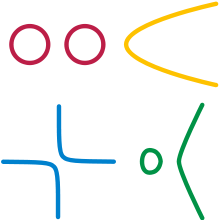Four manifolds from algebraic curves:
circles,  parabola,  hyperbola,  cubic.

Manifolds need not be connected (all in "one piece"); an example is a pair of separate circles. In this example we see that a manifold need not have any well-defined notion of distance, for there is no way to define the distance between points that don't lie in the same piece.

Manifolds need not be closed; thus a line segment without its end points is a manifold. And they are never countable, unless the dimension of the manifold is 0. Putting these freedoms together, other examples of manifolds are a parabola, a hyperbola (two open, infinite pieces) and the locus of points on a cubic curve y2 = x3x (a closed loop piece and an open, infinite piece).

However, we exclude examples like two touching circles that share a point to form a figure-8; at the shared point we cannot create a satisfactory chart. Even with the bending allowed by topology, the vicinity of the shared point looks like a "+", not a line (a + is not homeomorphic to a closed interval (line segment) since deleting the center point from the + gives a space with four components (i.e., pieces) whereas deleting a point from a closed interval gives a space with at most two pieces; topological operations always preserve the number of pieces).

### Enriched circle

Viewed using calculus, the circle transition function T is simply a function between open intervals, which gives a meaning to the statement that T is differentiable. The transition map T, and all the others, are differentiable on (0, 1); therefore, with this atlas the circle is a differentiable manifold. It is also smooth and analytic because the transition functions have these properties as well.

Other circle properties allow it to meet the requirements of more specialized types of manifold. For example, the circle has a notion of distance between two points, the arc-length between the points; hence it is a Riemannian manifold.

## History

The study of manifolds combines many important areas of mathematics: it generalizes concepts such as curves and surfaces as well as ideas from linear algebra and topology.

### Early development

Before the modern concept of a manifold there were several important results.

Non-Euclidean geometry considers spaces where Euclid's parallel postulate fails. Saccheri first studied them in 1733. Lobachevsky, Bolyai, and Riemann developed them 100 years later. Their research uncovered two types of spaces whose geometric structures differ from that of classical Euclidean space; these gave rise to hyperbolic geometry and elliptic geometry. In the modern theory of manifolds, these notions correspond to Riemannian manifolds with constant negative and positive curvature, respectively.

Carl Friedrich Gauss may have been the first to consider abstract spaces as mathematical objects in their own right. His theorema egregium gives a method for computing the curvature of a surface without considering the ambient space in which the surface lies. Such a surface would, in modern terminology, be called a manifold; and in modern terms, the theorem proved that the curvature of the surface is an intrinsic property. Manifold theory has come to focus exclusively on these intrinsic properties (or invariants), while largely ignoring the extrinsic properties of the ambient space.

Another, more topological example of an intrinsic property of a manifold is its Euler characteristic. Leonhard Euler showed that for a convex polytope in the three-dimensional Euclidean space with V vertices (or corners), E edges, and F faces,$V - E + F = 2.\$

The same formula will hold if we project the vertices and edges of the polytope onto a sphere, creating a topological map with V vertices, E edges, and F faces, and in fact, will remain true for any spherical map, even if it does not arise from any convex polytope. Thus 2 is a topological invariant of the sphere, called its Euler characteristic. On the other hand, a torus can be sliced open by its 'parallel' and 'meridian' circles, creating a map with V = 1 vertex, E = 2 edges, and F = 1 face. Thus the Euler characteristic of the torus is 1 − 2 + 1 = 0. The Euler characteristic of other surfaces is a useful topological invariant, which can be extended to higher dimensions using Betti numbers. In the mid nineteenth century, the Gauss–Bonnet theorem linked the Euler characteristic to the Gaussian curvature.

### Synthesis

Investigations of Niels Henrik Abel and Carl Gustav Jacobi on inversion of elliptic integrals in the first half of 19th century led them to consider special types of complex manifolds, now known as Jacobians. Bernhard Riemann further contributed to their theory, clarifying the geometric meaning of the process of analytic continuation of functions of complex variables.

Another important source of manifolds in 19th century mathematics was analytical mechanics, as developed by Simeon Poisson, Jacobi, and William Rowan Hamilton. The possible states of a mechanical system are thought to be points of an abstract space, phase space in Lagrangian and Hamiltonian formalisms of classical mechanics. This space is, in fact, a high-dimensional manifold, whose dimension corresponds to the degrees of freedom of the system and where the points are specified by their generalized coordinates. For an unconstrained movement of free particles the manifold is equivalent to the Euclidean space, but various conservation laws constrain it to more complicated formations, e.g. Liouville tori. The theory of a rotating solid body, developed in the 18th century by Leonhard Euler and Joseph Lagrange, gives another example where the manifold is nontrivial. Geometrical and topological aspects of classical mechanics were emphasized by Henri Poincaré, one of the founders of topology.

Riemann was the first one to do extensive work generalizing the idea of a surface to higher dimensions. The name manifold comes from Riemann's original German term, Mannigfaltigkeit, which William Kingdon Clifford translated as "manifoldness". In his Göttingen inaugural lecture, Riemann described the set of all possible values of a variable with certain constraints as a Mannigfaltigkeit, because the variable can have many values. He distinguishes between stetige Mannigfaltigkeit and diskrete Mannigfaltigkeit (continuous manifoldness and discontinuous manifoldness), depending on whether the value changes continuously or not. As continuous examples, Riemann refers to not only colors and the locations of objects in space, but also the possible shapes of a spatial figure. Using induction, Riemann constructs an n-fach ausgedehnte Mannigfaltigkeit (n times extended manifoldness or n-dimensional manifoldness) as a continuous stack of (n−1) dimensional manifoldnesses. Riemann's intuitive notion of a Mannigfaltigkeit evolved into what is today formalized as a manifold. Riemannian manifolds and Riemann surfaces are named after Riemann.

Hermann Weyl gave an intrinsic definition for differentiable manifolds in his lecture course on Riemann surfaces in 1911–1912, opening the road to the general concept of a topological space that followed shortly. During the 1930s Hassler Whitney and others clarified the foundational aspects of the subject, and thus intuitions dating back to the latter half of the 19th century became precise, and developed through differential geometry and Lie group theory. Notably, the Whitney embedding theorem showed that the intrinsic definition in terms of charts was equivalent to the extrinsic definition in terms of subsets of Euclidean space.

### Topology of manifolds: highlights

Two-dimensional manifolds, also known as a 2D surfaces embedded in our common 3D space, were considered by Riemann under the guise of Riemann surfaces, and rigorously classified in the beginning of the 20th century by Poul Heegaard and Max Dehn. Henri Poincaré pioneered the study of three-dimensional manifolds and raised a fundamental question about them, today known as the Poincaré conjecture. After nearly a century of effort by many mathematicians, starting with Poincaré himself, a consensus among experts (as of 2006) is that Grigori Perelman has proved the Poincaré conjecture (see the Solution of the Poincaré conjecture). Bill Thurston's geometrization program, formulated in the 1970s, provided a far-reaching extension of the Poincaré conjecture to the general three-dimensional manifolds. Four-dimensional manifolds were brought to the forefront of mathematical research in the 1980s by Michael Freedman and in a different setting, by Simon Donaldson, who was motivated by the then recent progress in theoretical physics (Yang-Mills theory), where they serve as a substitute for ordinary 'flat' space-time. Andrey Markov Jr. showed in 1960 that no algorithm exists for classifying four-dimensional manifolds. Important work on higher-dimensional manifolds, including analogues of the Poincaré conjecture, had been done earlier by René Thom, John Milnor, Stephen Smale and Sergei Novikov. One of the most pervasive and flexible techniques underlying much work on the topology of manifolds is Morse theory.

## Mathematical definition

Informally, a manifold is a space that is "modeled on" Euclidean space.

There are many different kinds of manifolds and generalizations. In geometry and topology, all manifolds are topological manifolds, possibly with additional structure, most often a differentiable structure. In terms of constructing manifolds via patching, a manifold has an additional structure if the transition maps between different patches satisfy axioms beyond just continuity. For instance, differentiable manifolds have homeomorphisms on overlapping neighborhoods diffeomorphic with each other, so that the manifold has a well-defined set of functions which are differentiable in each neighborhood, and so differentiable on the manifold as a whole.

Formally, a topological manifold is a second countable Hausdorff space that is locally homeomorphic to Euclidean space.

Second countable and Hausdorff are point-set conditions; second countable excludes spaces which are in some sense 'too large' such as the long line, while Hausdorff excludes spaces such as "the line with two origins" (these generalized manifolds are discussed in non-Hausdorff manifolds).

Locally homeomorphic to Euclidean space means that every point has a neighborhood homeomorphic to an open Euclidean n-ball,$\mathbf{B}^n = \{ (x_1, x_2, \dots, x_n)\in\mathbb{R}^n \mid x_1^2 + x_2^2 + \cdots + x_n^2 < 1 \}.$

Generally manifolds are taken to have a fixed dimension (the space must be locally homeomorphic to a fixed n-ball), and such a space is called an n-manifold; however, some authors admit manifolds where different points can have different dimensions. If a manifold has a fixed dimension, it is called a pure manifold. For example, the sphere has a constant dimension of 2 and is therefore a pure manifold whereas the disjoint union of a sphere and a line in three-dimensional space is not a pure manifold. Since dimension is a local invariant (i.e. the map sending each point to the dimension of its neighbourhood over which a chart is defined, is locally constant), each connected component has a fixed dimension.

Scheme-theoretically, a manifold is a locally ringed space, whose structure sheaf is locally isomorphic to the sheaf of continuous (or differentiable, or complex-analytic, etc.) functions on Euclidean space. This definition is mostly used when discussing analytic manifolds in algebraic geometry.

The broadest common definition of manifold is a topological space locally homeomorphic to a topological vector space over the reals. This omits the point-set axioms, allowing higher cardinalities and non-Hausdorff manifolds; and it omits finite dimension, allowing structures such as Hilbert manifolds to be modeled on Hilbert spaces, Banach manifolds to be modeled on Banach spaces, and Fréchet manifolds to be modeled on Fréchet spaces. Usually one relaxes one or the other condition: manifolds with the point-set axioms are studied in general topology, while infinite-dimensional manifolds are studied in functional analysis.

## Charts, atlases, and transition maps

For more exact definitions, see Differentiable manifold#Definition.

The spherical Earth is navigated using flat maps or charts, collected in an atlas. Similarly, a differentiable manifold can be described using mathematical maps, called coordinate charts, collected in a mathematical atlas. It is not generally possible to describe a manifold with just one chart, because the global structure of the manifold is different from the simple structure of the charts. For example, no single flat map can represent the entire Earth without separation of adjacent features across the map's boundaries or duplication of coverage. When a manifold is constructed from multiple overlapping charts, the regions where they overlap carry information essential to understanding the global structure.

### Charts

A coordinate map, a coordinate chart, or simply a chart, of a manifold is an invertible map between a subset of the manifold and a simple space such that both the map and its inverse preserve the desired structure. For a topological manifold, the simple space is some Euclidean space Rn and interest focuses on the topological structure. This structure is preserved by homeomorphisms, invertible maps that are continuous in both directions.

In the case of a differentiable manifold, a set of charts called an atlas allows us to do calculus on manifolds. Polar coordinates, for example, form a chart for the plane R2 minus the positive x-axis and the origin. Another example of a chart is the map χtop mentioned in the section above, a chart for the circle.

### Atlases

The description of most manifolds requires more than one chart (a single chart is adequate for only the simplest manifolds). A specific collection of charts which covers a manifold is called an atlas. An atlas is not unique as all manifolds can be covered multiple ways using different combinations of charts. Two atlases are said to be Ck equivalent if their union is also a Ck atlas.

The atlas containing all possible charts consistent with a given atlas is called the maximal atlas (i.e. an equivalence class containing that given atlas (under the already defined equivalence relation given in the previous paragraph)). Unlike an ordinary atlas, the maximal atlas of a given manifold is unique. Though it is useful for definitions, it is an abstract object and not used directly (e.g. in calculations).

### Transition maps

Charts in an atlas may overlap and a single point of a manifold may be represented in several charts. If two charts overlap, parts of them represent the same region of the manifold, just as a map of Europe and a map of Asia may both contain Moscow. Given two overlapping charts, a transition function can be defined which goes from an open ball in Rn to the manifold and then back to another (or perhaps the same) open ball in Rn. The resultant map, like the map T in the circle example above, is called a change of coordinates, a coordinate transformation, a transition function, or a transition map.

An atlas can also be used to define additional structure on the manifold. The structure is first defined on each chart separately. If all the transition maps are compatible with this structure, the structure transfers to the manifold.

This is the standard way differentiable manifolds are defined. If the transition functions of an atlas for a topological manifold preserve the natural differential structure of Rn (that is, if they are diffeomorphisms), the differential structure transfers to the manifold and turns it into a differentiable manifold. Complex manifolds are introduced in an analogous way by requiring that the transition functions of an atlas are holomorphic functions. For symplectic manifolds, the transition functions must be symplectomorphisms.

The structure on the manifold depends on the atlas, but sometimes different atlases can be said to give rise to the same structure. Such atlases are called compatible.

These notions are made precise in general through the use of pseudogroups.

## Construction

A single manifold can be constructed in different ways, each stressing a different aspect of the manifold, thereby leading to a slightly different viewpoint.

### Charts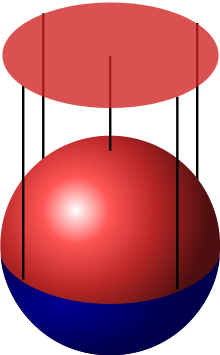The chart maps the part of the sphere with positive z coordinate to a disc.

Perhaps the simplest way to construct a manifold is the one used in the example above of the circle. First, a subset of R2 is identified, and then an atlas covering this subset is constructed. The concept of manifold grew historically from constructions like this. Here is another example, applying this method to the construction of a sphere:

#### Sphere with charts

A sphere can be treated in almost the same way as the circle. In mathematics a sphere is just the surface (not the solid interior), which can be defined as a subset of R3:$S = \{ (x,y,z) \in \mathbf{R}^3 | x^2 + y^2 + z^2 = 1 \}.$

The sphere is two-dimensional, so each chart will map part of the sphere to an open subset of R2. Consider the northern hemisphere, which is the part with positive z coordinate (coloured red in the picture on the right). The function χ defined by$\chi(x,y,z) = (x,y),\$

maps the northern hemisphere to the open unit disc by projecting it on the (x, y) plane. A similar chart exists for the southern hemisphere. Together with two charts projecting on the (x, z) plane and two charts projecting on the (y, z) plane, an atlas of six charts is obtained which covers the entire sphere.

This can be easily generalized to higher-dimensional spheres.

### Patchwork

A manifold can be constructed by gluing together pieces in a consistent manner, making them into overlapping charts. This construction is possible for any manifold and hence it is often used as a characterisation, especially for differentiable and Riemannian manifolds. It focuses on an atlas, as the patches naturally provide charts, and since there is no exterior space involved it leads to an intrinsic view of the manifold.

The manifold is constructed by specifying an atlas, which is itself defined by transition maps. A point of the manifold is therefore an equivalence class of points which are mapped to each other by transition maps. Charts map equivalence classes to points of a single patch. There are usually strong demands on the consistency of the transition maps. For topological manifolds they are required to be homeomorphisms; if they are also diffeomorphisms, the resulting manifold is a differentiable manifold.

This can be illustrated with the transition map t = 1s from the second half of the circle example. Start with two copies of the line. Use the coordinate s for the first copy, and t for the second copy. Now, glue both copies together by identifying the point t on the second copy with the point s = 1t on the first copy (the points t = 0 and s = 0 are not identified with any point on the first and second copy, respectively). This gives a circle.

#### Intrinsic and extrinsic view

The first construction and this construction are very similar, but they represent rather different points of view. In the first construction, the manifold is seen as embedded in some Euclidean space. This is the extrinsic view. When a manifold is viewed in this way, it is easy to use intuition from Euclidean spaces to define additional structure. For example, in a Euclidean space it is always clear whether a vector at some point is tangential or normal to some surface through that point.

The patchwork construction does not use any embedding, but simply views the manifold as a topological space by itself. This abstract point of view is called the intrinsic view. It can make it harder to imagine what a tangent vector might be, and there is no intrinsic notion of a normal bundle, but instead there is an intrinsic stable normal bundle.

#### n-Sphere as a patchwork

The n-sphere Sn is a generalisation of the idea of a circle (1-sphere) and sphere (2-sphere) to higher dimensions. An n-sphere Sn can be constructed by gluing together two copies of Rn. The transition map between them is defined as$\mathbf{R}^n \setminus \{0\} \to \mathbf{R}^n \setminus \{0\}: x \mapsto x/\|x\|^2.$

This function is its own inverse and thus can be used in both directions. As the transition map is a smooth function, this atlas defines a smooth manifold. In the case n = 1, the example simplifies to the circle example given earlier.

### Identifying points of a manifold

It is possible to define different points of a manifold to be same. This can be visualized as gluing these points together in a single point, forming a quotient space. There is, however, no reason to expect such quotient spaces to be manifolds. Among the possible quotient spaces that are not necessarily manifolds, orbifolds and CW complexes are considered to be relatively well-behaved. An example of a quotient space of a manifold that is also a manifold is the real projective space identified as a quotient space of the corresponding sphere.

One method of identifying points (gluing them together) is through a right (or left) action of a group, which acts on the manifold. Two points are identified if one is moved onto the other by some group element. If M is the manifold and G is the group, the resulting quotient space is denoted by M / G (or G \ M).

Manifolds which can be constructed by identifying points include tori and real projective spaces (starting with a plane and a sphere, respectively).

### Manifold with boundary

A manifold with boundary is a manifold with an edge. For example a sheet of paper with rounded corners is a 2-manifold with a 1-dimensional boundary. The boundary of an n-manifold with boundary is an (n − 1)-manifold. A disk (circle plus interior) is a 2-manifold with boundary. Its boundary is a circle, a 1-manifold. A ball (sphere plus interior) is a 3-manifold with boundary. Its boundary is a sphere, a 2-manifold. (See also Boundary (topology)).

In technical language, a manifold with boundary is a space containing both interior points and boundary points. Every interior point has a neighborhood homeomorphic to the open n-ball {(x1x2, …, xn) | Σ xi2 < 1}. Every boundary point has a neighborhood homeomorphic to the "half" n-ball {(x1x2, …, xn) | Σ xi2 < 1 and x1 ≥ 0}. The homeomorphism must send the boundary point to a point with x1 = 0.

### Gluing along boundaries

Two manifolds with boundaries can be glued together along a boundary. If this is done the right way, the result is also a manifold. Similarly, two boundaries of a single manifold can be glued together.

Formally, the gluing is defined by a bijection between the two boundaries[dubious ]. Two points are identified when they are mapped onto each other. For a topological manifold this bijection should be a homeomorphism, otherwise the result will not be a topological manifold. Similarly for a differentiable manifold it has to be a diffeomorphism. For other manifolds other structures should be preserved.

A finite cylinder may be constructed as a manifold by starting with a strip [0, 1] × [0, 1] and gluing a pair of opposite edges on the boundary by a suitable diffeomorphism. A projective plane may be obtained by gluing a sphere with a hole in it to a Möbius strip along their respective circular boundaries.

### Cartesian products

The Cartesian product of manifolds is also a manifold.

The dimension of the product manifold is the sum of the dimensions of its factors. Its topology is the product topology, and a Cartesian product of charts is a chart for the product manifold. Thus, an atlas for the product manifold can be constructed using atlases for its factors. If these atlases define a differential structure on the factors, the corresponding atlas defines a differential structure on the product manifold. The same is true for any other structure defined on the factors. If one of the factors has a boundary, the product manifold also has a boundary. Cartesian products may be used to construct tori and finite cylinders, for example, as S1 × S1 and S1 × [0, 1], respectively.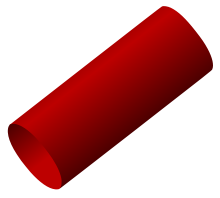A finite cylinder is a manifold with boundary.

### Topological manifolds

The simplest kind of manifold to define is the topological manifold, which looks locally like some "ordinary" Euclidean space Rn. Formally, a topological manifold is a topological space locally homeomorphic to a Euclidean space. This means that every point has a neighbourhood for which there exists a homeomorphism (a bijective continuous function whose inverse is also continuous) mapping that neighbourhood to Rn. These homeomorphisms are the charts of the manifold.

It is to be noted that a topological manifold looks locally like a Euclidean space in a rather weak manner: while for each individual chart it is possible to distinguish differentiable functions or measure distances and angles, merely by virtue of being a topological manifold a space does not have any particular and consistent choice of such concepts. In order to discuss such properties for a manifold, one needs to specify further structure and consider differentiable manifolds and Riemannian manifolds discussed below. In particular, the same underlying topological manifold can have several mutually incompatible classes of differentiable functions and an infinite number of ways to specify distances and angles.

Usually additional technical assumptions on the topological space are made to exclude pathological cases. It is customary to require that the space be Hausdorff and second countable.

The dimension of the manifold at a certain point is the dimension of the Euclidean space that the charts at that point map to (number n in the definition). All points in a connected manifold have the same dimension. Some authors require that all charts of a topological manifold map to Euclidean spaces of same dimension. In that case every topological manifold has a topological invariant, its dimension. Other authors allow disjoint unions of topological manifolds with differing dimensions to be called manifolds.

### Differentiable manifolds

For most applications a special kind of topological manifold, a differentiable manifold, is used. If the local charts on a manifold are compatible in a certain sense, one can define directions, tangent spaces, and differentiable functions on that manifold. In particular it is possible to use calculus on a differentiable manifold. Each point of an n-dimensional differentiable manifold has a tangent space. This is an n-dimensional Euclidean space consisting of the tangent vectors of the curves through the point.

Two important classes of differentiable manifolds are smooth and analytic manifolds. For smooth manifolds the transition maps are smooth, that is infinitely differentiable. Analytic manifolds are smooth manifolds with the additional condition that the transition maps are analytic (they can be expressed as power series, which are essentially polynomials of infinite degree). The sphere can be given analytic structure, as can most familiar curves and surfaces.

A rectifiable set generalizes the idea of a piecewise smooth or rectifiable curve to higher dimensions; however, rectifiable sets are not in general manifolds.

### Riemannian manifolds

To measure distances and angles on manifolds, the manifold must be Riemannian. A Riemannian manifold is a differentiable manifold in which each tangent space is equipped with an inner product 〈⋅,⋅〉 in a manner which varies smoothly from point to point. Given two tangent vectors u and v, the inner product〈u,v〉gives a real number. The dot (or scalar) product is a typical example of an inner product. This allows one to define various notions such as length, angles, areas (or volumes), curvature, gradients of functions and divergence of vector fields.

All differentiable manifolds (of constant dimension) can be given the structure of a Riemannian manifold. The Euclidean space itself carries a natural structure of Riemannian manifold (the tangent spaces are naturally identified with the Euclidean space itself and carry the standard scalar product of the space). Many familiar curves and surfaces, including for example all n-spheres, are specified as subspaces of a Euclidean space and inherit a metric from their embedding in it.

### Finsler manifolds

A Finsler manifold allows the definition of distance but does not require the concept of angle; it is an analytic manifold in which each tangent space is equipped with a norm, ||·||, in a manner which varies smoothly from point to point. This norm can be extended to a metric, defining the length of a curve; but it cannot in general be used to define an inner product.

Any Riemannian manifold is a Finsler manifold.

### Lie groups

Lie groups, named after Sophus Lie, are differentiable manifolds that carry also the structure of a group which is such that the group operations are defined by smooth maps.

A Euclidean vector space with the group operation of vector addition is an example of a non-compact Lie group. A simple example of a compact Lie group is the circle: the group operation is simply rotation. This group, known as U(1), can be also characterised as the group of complex numbers of modulus 1 with multiplication as the group operation. Other examples of Lie groups include special groups of matrices, which are all subgroups of the general linear group, the group of n by n matrices with non-zero determinant. If the matrix entries are real numbers, this will be an n2-dimensional disconnected manifold. The orthogonal groups, the symmetry groups of the sphere and hyperspheres, are n(n-1)/2 dimensional manifolds, where n-1 is the dimension of the sphere. Further examples can be found in the table of Lie groups.

### Other types of manifolds

• A complex manifold is a manifold modeled on Cn with holomorphic transition functions on chart overlaps. These manifolds are the basic objects of study in complex geometry. A one-complex-dimensional manifold is called a Riemann surface. Note that an n-dimensional complex manifold has dimension 2n as a real differentiable manifold.
• A CR manifold is a manifold modeled on boundaries of domains in Cn.
• Infinite dimensional manifolds: to allow for infinite dimensions, one may consider Banach manifolds which are locally homeomorphic to Banach spaces. Similarly, Fréchet manifolds are locally homeomorphic to Fréchet spaces.
• A symplectic manifold is a kind of manifold which is used to represent the phase spaces in classical mechanics. They are endowed with a 2-form that defines the Poisson bracket. A closely related type of manifold is a contact manifold.
• A combinatorial manifold is a kind of manifold which is discretization of a manifold. It usually means a piecewise linear manifold made by simplicial complexes.
• A digital manifold is a special kind of combinatorial manifold which is defined in digital space. See digital topology

## Classification and invariants

Different notions of manifolds have different notions of classification and invariant; in this section we focus on smooth closed manifolds.

The classification of smooth closed manifolds is well-understood in principle, except in dimension 4: in low dimensions (2 and 3) it is geometric, via the uniformization theorem and the Solution of the Poincaré conjecture, and in high dimension (5 and above) it is algebraic, via surgery theory. This is a classification in principle: the general question of whether two smooth manifolds are diffeomorphic is not computable in general. Further, specific computations remain difficult, and there are many open questions.

Orientable surfaces can be visualized, and their diffeomorphism classes enumerated, by genus. Given two orientable surfaces, one can determine if they are diffeomorphic by computing their respective genera and comparing: they are diffeomorphic if and only if the genera are equal, so the genus forms a complete set of invariants.

This is much harder in higher dimensions: higher dimensional manifolds cannot be directly visualized (though visual intuition is useful in understanding them), nor can their diffeomorphism classes be enumerated, nor can one in general determine if two different descriptions of a higher-dimensional manifold refer to the same object.

However, one can determine if two manifolds are different if there is some intrinsic characteristic that differentiates them. Such criteria are commonly referred to as invariants, because, while they may be defined in terms of some presentation (such as the genus in terms of a triangulation), they are the same relative to all possible descriptions of a particular manifold: they are invariant under different descriptions.

Naively, one could hope to develop an arsenal of invariant criteria that would definitively classify all manifolds up to isomorphism. Unfortunately, it is known that for manifolds of dimension 4 and higher, no program exists that can decide whether two manifolds are diffeomorphic.

Smooth manifolds have a rich set of invariants, coming from point-set topology, classic algebraic topology, and geometric topology. The most familiar invariants, which are visible for surfaces, are orientability (a normal invariant, also detected by homology) and genus (a homological invariant).

Smooth closed manifolds have no local invariants (other than dimension), though geometric manifolds have local invariants, notably the curvature of a Riemannian manifold and the torsion of a manifold equipped with an affine connection. This distinction between no local invariants and local invariants is a common way to distinguish between geometry and topology. All invariants of a smooth closed manifold are thus global.

Algebraic topology is a source of a number of important global invariant properties. Some key criteria include the simply connected property and orientability (see below). Indeed several branches of mathematics, such as homology and homotopy theory, and the theory of characteristic classes were founded in order to study invariant properties of manifolds.

## Examples of surfaces

### Orientability

In dimensions two and higher, a simple but important invariant criterion is the question of whether a manifold admits a meaningful orientation. Consider a topological manifold with charts mapping to Rn. Given an ordered basis for Rn, a chart causes its piece of the manifold to itself acquire a sense of ordering, which in 3-dimensions can be viewed as either right-handed or left-handed. Overlapping charts are not required to agree in their sense of ordering, which gives manifolds an important freedom. For some manifolds, like the sphere, charts can be chosen so that overlapping regions agree on their "handedness"; these are orientable manifolds. For others, this is impossible. The latter possibility is easy to overlook, because any closed surface embedded (without self-intersection) in three-dimensional space is orientable.

Some illustrative examples of non-orientable manifolds include: (1) the Möbius strip, which is a manifold with boundary, (2) the Klein bottle, which must intersect itself in 3-space, and (3) the real projective plane, which arises naturally in geometry.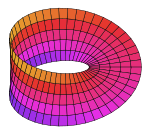Möbius strip

#### Möbius strip

Begin with an infinite circular cylinder standing vertically, a manifold without boundary. Slice across it high and low to produce two circular boundaries, and the cylindrical strip between them. This is an orientable manifold with boundary, upon which "surgery" will be performed. Slice the strip open, so that it could unroll to become a rectangle, but keep a grasp on the cut ends. Twist one end 180°, making the inner surface face out, and glue the ends back together seamlessly. This results in a strip with a permanent half-twist: the Möbius strip. Its boundary is no longer a pair of circles, but (topologically) a single circle; and what was once its "inside" has merged with its "outside", so that it now has only a single side.

#### Klein bottle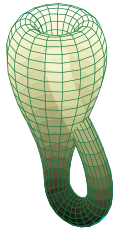The Klein bottle immersed in three-dimensional space.

Take two Möbius strips; each has a single loop as a boundary. Straighten out those loops into circles, and let the strips distort into cross-caps. Gluing the circles together will produce a new, closed manifold without boundary, the Klein bottle. Closing the surface does nothing to improve the lack of orientability, it merely removes the boundary. Thus, the Klein bottle is a closed surface with no distinction between inside and outside. Note that in three-dimensional space, a Klein bottle's surface must pass through itself. Building a Klein bottle which is not self-intersecting requires four or more dimensions of space.

#### Real projective plane

Begin with a sphere centered on the origin. Every line through the origin pierces the sphere in two opposite points called antipodes. Although there is no way to do so physically, it is possible to mathematically merge each antipode pair into a single point. The closed surface so produced is the real projective plane, yet another non-orientable surface. It has a number of equivalent descriptions and constructions, but this route explains its name: all the points on any given line through the origin project to the same "point" on this "plane".

### Genus and the Euler characteristic

For two dimensional manifolds a key invariant property is the genus, or the "number of handles" present in a surface. A torus is a sphere with one handle, a double torus is a sphere with two handles, and so on. Indeed it is possible to fully characterize compact, two-dimensional manifolds on the basis of genus and orientability. In higher-dimensional manifolds genus is replaced by the notion of Euler characteristic, and more generally Betti numbers and homology and cohomology.

## Maps of manifolds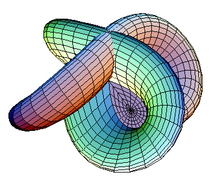A Morin surface, an immersion used in sphere eversion.

Just as there are various types of manifolds, there are various types of maps of manifolds. In addition to continuous functions and smooth functions generally, there are maps with special properties. In geometric topology a basic type are embeddings, of which knot theory is a central example, and generalizations such as immersions, submersions, covering spaces, and ramified covering spaces. Basic results include the Whitney embedding theorem and Whitney immersion theorem.

In Riemannian geometry, one may ask for maps to preserve the Riemannian metric, leading to notions of isometric embeddings, isometric immersions, and Riemannian submersions; a basic result is the Nash embedding theorem.

### Scalar-valued functions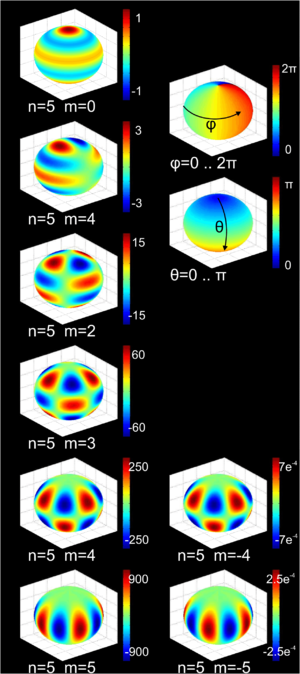3D color plot of the spherical harmonics of degree n = 5

A basic example of maps between manifolds are scalar-valued functions on a manifold,$f\colon M \to \mathbf{R}$ or$f\colon M \to \mathbf{C},$

sometimes called regular functions or functionals, by analogy with algebraic geometry or linear algebra. These are of interest both in their own right, and to study the underlying manifold.

In geometric topology, most commonly studied are Morse functions, which yield handlebody decompositions, while in mathematical analysis, one often studies solution to partial differential equations, an important example of which is harmonic analysis, where one studies harmonic functions: the kernel of the Laplace operator. This leads to such functions as the spherical harmonics, and to heat kernel methods of studying manifolds, such as hearing the shape of a drum and some proofs of the Atiyah–Singer index theorem.

## Generalizations of manifolds

• Orbifolds: An orbifold is a generalization of manifold allowing for certain kinds of "singularities" in the topology. Roughly speaking, it is a space which locally looks like the quotients of some simple space (e.g. Euclidean space) by the actions of various finite groups. The singularities correspond to fixed points of the group actions, and the actions must be compatible in a certain sense.
• Algebraic varieties and schemes: Non-singular algebraic varieties over the real or complex numbers are manifolds. One generalizes this first by allowing singularities, secondly by allowing different fields, and thirdly by emulating the patching construction of manifolds: just as a manifold is glued together from open subsets of Euclidean space, an algebraic variety is glued together from affine algebraic varieties, which are zero sets of polynomials over algebraically closed fields. Schemes are likewise glued together from affine schemes, which are a generalization of algebraic varieties. Both are related to manifolds, but are constructed algebraically using sheaves instead of atlases.
Because of singular points, a variety is in general not a manifold, though linguistically the French variété, German Mannigfaltigkeit and English manifold are largely synonymous. In French an algebraic variety is called une variété algébrique (an algebraic variety), while a smooth manifold is called une variété différentielle (a differential variety).
• CW-complexes: A CW complex is a topological space formed by gluing disks of different dimensionality together. In general the resulting space is singular, and hence not a manifold. However, they are of central interest in algebraic topology, especially in homotopy theory, as they are easy to compute with and singularities are not a concern.

### By dimension

Wikimedia Foundation. 2010.

Synonyms:

### Look at other dictionaries:

• manifold — [ manifɔld ] n. m. • 1930; mot angl., pour manifold paper ♦ Anglic. 1 ♦ Carnet comportant plusieurs séries de feuilles et de papier carbone. 2 ♦ (1960) Techn. Ensemble de vannes et de conduits orientant un fluide vers un réservoir ou des… …   Encyclopédie Universelle

• manifold — [man′ə fōld΄] adj. [ME < OE manigfeald: see MANY & FOLD] 1. having many and various forms, features, parts, etc. [manifold wisdom] 2. of many sorts; many and varied; multifarious: used with a plural noun [manifold duties] 3. being such in many …   English World dictionary

• Manifold — Man i*fold, a. [AS. manigfeald. See {Many}, and {Fold}.] 1. Various in kind or quality; many in number; numerous; multiplied; complicated. [1913 Webster] O Lord, how manifold are thy works! Ps. civ. 24. [1913 Webster] I know your manifold… …   The Collaborative International Dictionary of English

• manifold — manifóld s. n., pl. manifólde Trimis de siveco, 10.08.2004. Sursa: Dicţionar ortografic ﻿ MANIFÓLD s.n. 1. (Anglicism) Carnet de note, de facturi etc. cu cotor. 2. (tehn.) Colector; conductă de aducţie. ♦ Claviatură. [< engl., fr. manifold] …   Dicționar Român

• Manifold — Man i*fold, n. 1. A copy of a writing made by the manifold process. [1913 Webster] 2. (Mech.) A cylindrical pipe fitting, having a number of lateral outlets, for connecting one pipe with several others; as, the exhaust manifold of an automobile… …   The Collaborative International Dictionary of English

• Manifold — Man i*fold, v. t. [imp. & p. p. {Manifolded}; p. pr. & vb. n. {Manifolding}.] To take copies of by the process of manifold writing; as, to manifold a letter. [1913 Webster] …   The Collaborative International Dictionary of English

• manifold — I adjective assorted, considerable, divers, diverse, diversified, innumerable, many, miscellaneous, multifarious, multifold, multiform, multiple, multiplex, multiplicate, multitudinous, myriad, numerous, populous, profuse, several, sundry,… …   Law dictionary

• Manifold —   [ mænɪfəʊld], John Streeter, australischer Schriftsteller und Musikhistoriker, * Melbourne 21. 4. 1915, ✝ Brisbane 19. 4. 1985; verfasste v. a. satirische, aber auch romantische Verse sowie Lyrik über den Krieg. Bekannt wurde er auch als… …   Universal-Lexikon

• manifold — [adj] abundant, many assorted, complex, copious, different, diverse, diversified, diversiform, multifarious, multifold, multiform, multiple, multiplied, multitudinous, multivarious, numerous, sundry, varied, various; concepts 564,762,781 Ant. one …   New thesaurus

• manifold — ► ADJECTIVE formal or literary 1) many and various. 2) having many different forms. ► NOUN 1) a pipe or chamber branching into several openings. 2) (in an internal combustion engine) the part conveying air and fuel from the carburettor to the… …   English terms dictionary